# mesh

Mesh properties of metal or dielectric antenna or array structure

## Syntax

``mesh(object)``
``mesh(shape)``
``mesh(object,Name,Value)``
``meshdata = mesh(___,Name,Value)``

## Description

````mesh(object)` plots the mesh used to analyze antenna or array element.```

example

````mesh(shape)` plots the mesh for the shapes.```

example

````mesh(object,Name,Value)` changes and plots the mesh structure of an antenna or array element, using additional options specified by the name-value pairs. You can also determine the number of unknowns from the number of basis functions in the output.```

example

````meshdata = mesh(___,Name,Value)` returns a mesh structure that specifies the properties used to analyze the antenna or array.```

## Examples

collapse all

Create and view the mesh structure of a top-hat monopole antenna with Maximum edge length of 0.1 m.

```h = monopoleTopHat; i = impedance(h,75e6)```
```i = 2.4349e+02 + 5.9132e+02i ```
`mesh(h)`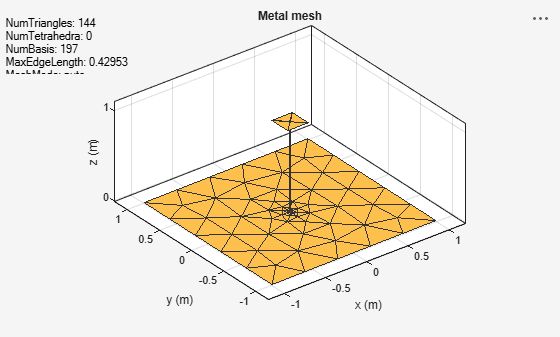`m = mesh(h)`
```m = struct with fields: NumTriangles: 148 NumTetrahedra: 0 NumBasis: 203 MaxEdgeLength: 0.4295 MeshMode: 'auto' ```

Radiation Pattern of Microstrip Patch Antenna

Create a microstrip patch antenna using 'FR4' as the dielectric substrate.

```d = dielectric('FR4'); pm = patchMicrostrip('Length',75e-3, 'Width',37e-3, ... 'GroundPlaneLength',120e-3, 'GroundPlaneWidth',120e-3, ... 'Substrate',d); show(pm)```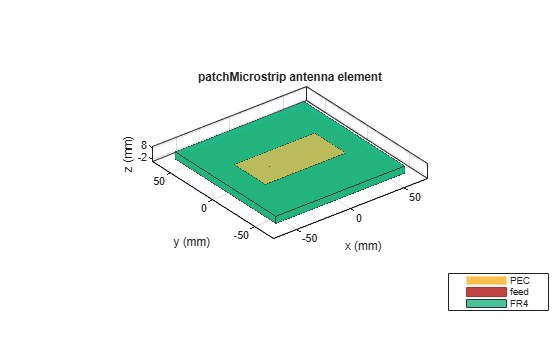Plot the radiation pattern of the antenna at a frequency of 1.67 GHz.

```figure pattern(pm,1.67e9)```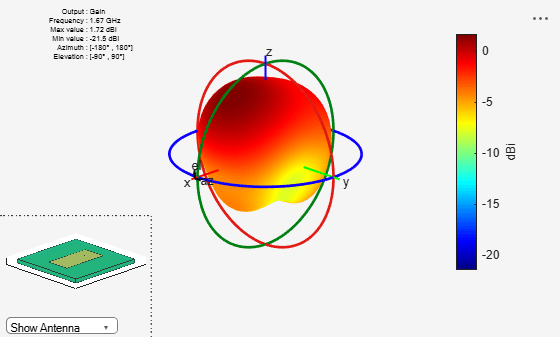Mesh the whole antenna.

```figure mesh(pm)```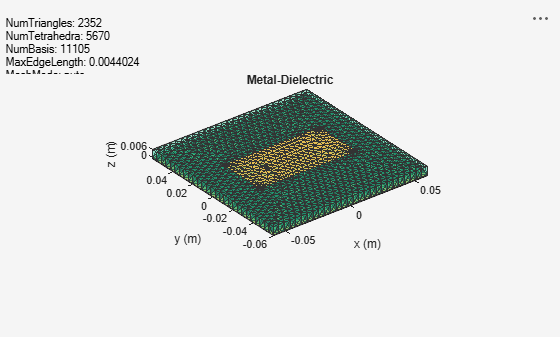Mesh only the dielectric surface of the antenna.

```figure mesh(pm,'View','dielectric surface')```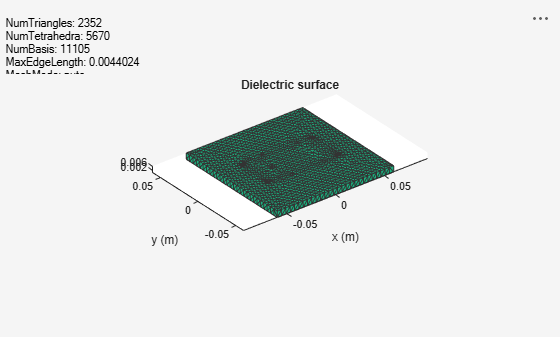Create a rectangular and circular shape, intersect them and mesh at a wavelength of 2 m.

```r = antenna.Rectangle; c = antenna.Circle; p = r&c; mesh(p,2);```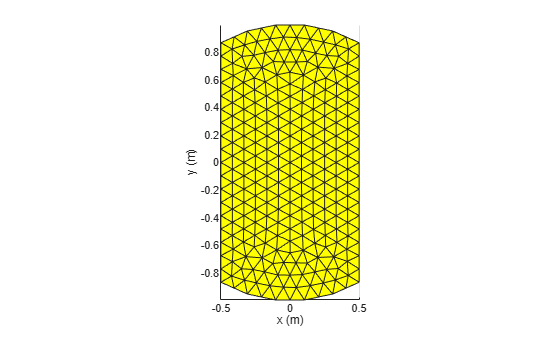Create a default horn antenna. Mesh the structure with the `'Slicer'` argument set to `'on'`.

```ant = horn; z = impedance(ant,70e6); mesh(ant,'Slicer','on')```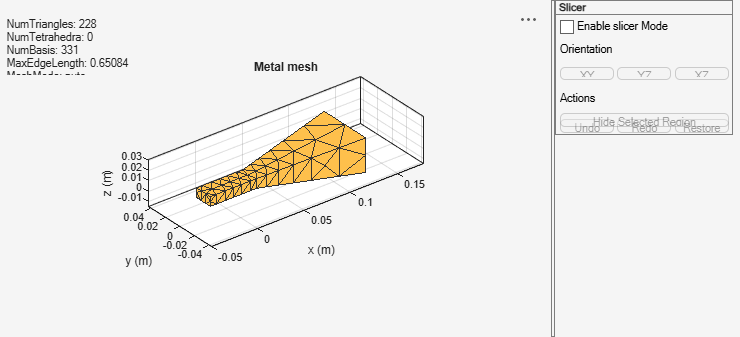Select Enable slicer Mode. Then select a plane for the slice under Orientation.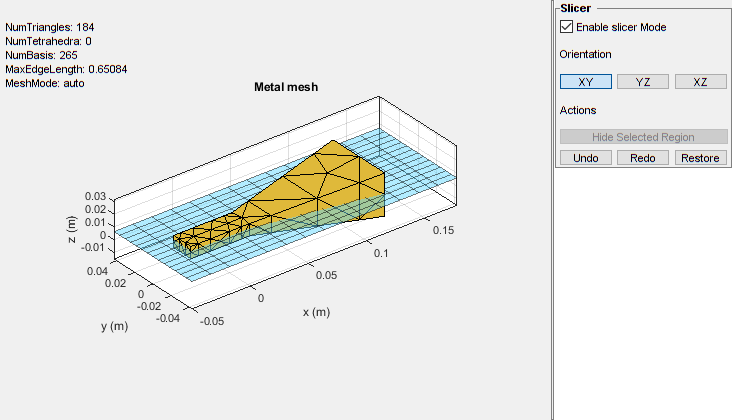Click on the plot and select a region to hide.Click Hide Selected Region to view the desired slice.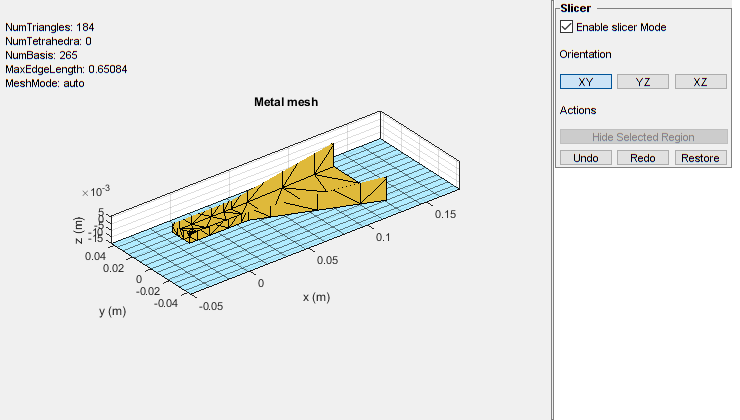## Input Arguments

collapse all

Antenna or array element, specified as an object.

Shape created using custom elements and shape objects of Antenna Toolbox, specified as an object. You can create the shapes using `antenna.Circle`, `antenna.Polygon`, or `antenna.Rectangle`.

Example: `c = antenna.Rectangle; mesh(c)`

### Name-Value Arguments

Example: ‘MaxEdgeLength’, 0.1

Specify optional comma-separated pairs of `Name,Value` pair arguments. `Name` is the argument name and `Value` is the corresponding value. `Name` must appear inside single quotes (`''`). You can specify several name and value pair arguments in any order as ```Name1, Value1, ..., NameN, ValueN```.

Maximum edge length of triangles in mesh, specified as a comma-separated pair consisting of `'MaxEdgeLength'` and a scalar in meters. All triangles in the mesh have sides less than or equal to the `'MaxEdgeLength'` value.

Data Types: `double`

Minimum edge length of triangles in mesh, specified as a comma-separated pair consisting of `'MinEdgeLength'` and a scalar in meters. All triangles in the mesh have sides greater than or equal to the `'MinEdgeLength'`.

Note

You can use this property only with the `pcbStack` object.

Data Types: `double`

Mesh growth rate, specified as a comma-separated pair consisting of `'GrowthRate'` and a scalar. The default value of `0.7` states that the growth rate of the mesh is 70 percent. Growth rate values lie between `0` and `1`.

Note

You can use this property only with the `pcbStack` object.

Data Types: `double`

Customize mesh view of antenna or array element, specified as a comma-separated pair consisting of `'View'` and `'all'`, `'metal'`,`'dielectric surface'`, or `'dielectric volume'`.

You choose `'dielectric surface'` to view the boundary triangle mesh of the dielectric. You choose `'dielectric volume'` to view the tetrahedral volume mesh of the dielectric.

Data Types: `char`

Flag to enable or disable plot interactivity, specified as `'on'` or `'off'`, or as numeric or logical `1(true)` or `0(false)`. Setting this flag to `1` or `'on'` opens a plot with the Slicer panel, where you can slice and view the desired cross section of the plot along the XY-, YZ-, and XZ- planes. Setting this flag to `0` or `'off'` opens a plot without the Slicer panel.

Data Types: `string` | `logical`

## Version History

Introduced in R2015a